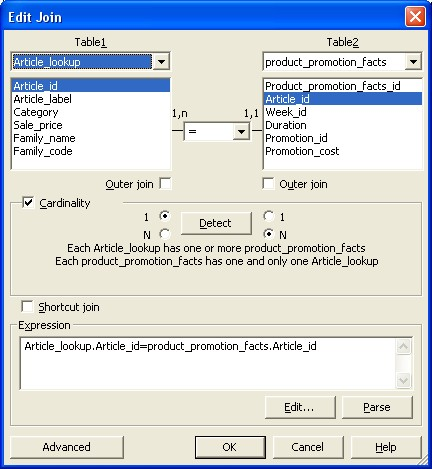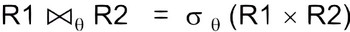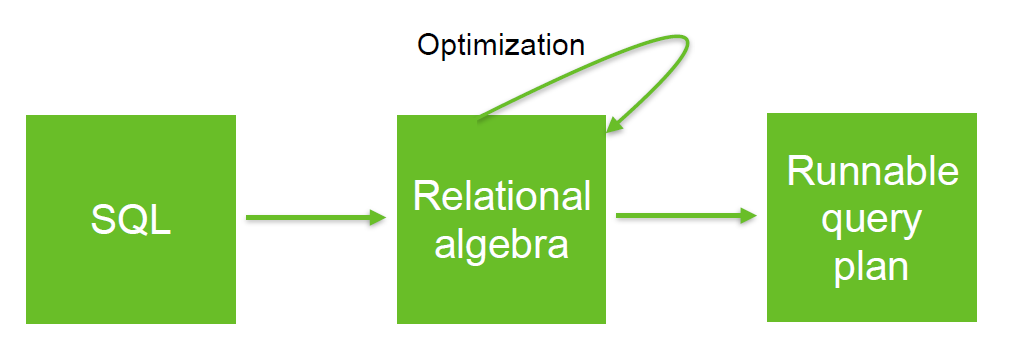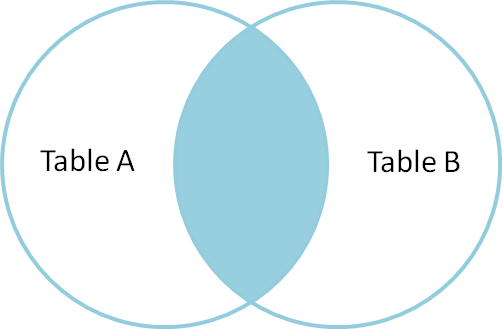# Relational Operator - Equi-joins

An Equi-join is a join where the condition (predicate) is an equality.

All other join operations with a predicate other than equality are called theta join

## Syntax

An equi-join links two relations (tables, …) on common values in a column in relation 1 with a column in relation 2.

### Conditional

The restriction conforms to the following syntax when the condition is specified:

• Relational Syntax

$$\LARGE R \bowtie_{R.A = S.A} S$$

• SQL
Table1.column_a = Table2.column_a


### Natural

A natural join is a shorthand equi-join where the equality applied to all shared attributes.

$$\LARGE R \bowtie_{attrs(R) \cap attrs(S)} S$$ where:

• $attrs(R) \cap attrs(S)$ is the intersection of the attributes of R and S.

## Rule

$$\LARGE R_1 \bowtie_{ a = b } R_2 = \sigma_{a=b} ( R_1 \times R_2 )$$

The join of R1 and R2 on A=B is the same that the selection of the cross product of R1 and R2 where A=B.

## Notation

### Sql Notation

Discover MoreEqui-joins in BOBJ

in Bobj. You have two equi-join types in : the simple the complex (This is a single join that links multiple columns between two tables. ) You can create a complex equi-join. This is a single...Relational Algebra - Theta-join

A theta-join is a difficult/complex join where the condition is not a equality. Example: Band join or range join. A theta is a join that links tables based on a relationship other than the equality...Relational Operator - Join

join is a relational operator that pair tuples (row) of two relations (table, ...) according to a condition (predicate). where: c is a condition (ie predicate) See Type Symbol Unicode...SQL - (Equi|Simple|Inner|Natural) Join

in SQL An equi-join also known as simple join link relation (tables, ...) based on the equality between the values in the column of one relation (table, ...) and the values in the column of another. Because...